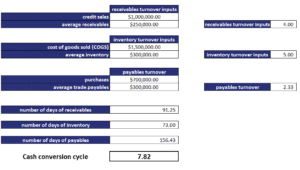# Cash conversion cycle

The cash conversion cycle (CCC) or net operating cycle is an important concept that measure how efficiently a company is using its working capital management. In particular, the CCC is the number of days it takes to turn the firm’s cash investment in inventory back in to cash, in the form of cash receipts from the sale of that inventory. This is different from the operating cycle, which only tells us the average number of days it takes a company to turn raw materials into cash proceeds from sales.

On this page, we discuss the CCC definition, report the different components needed, and discuss how to interpret and compare the CCC among different companies. At the bottom of this page, we include a cash conversion cycle Excel spreadsheet that is available for download.

# Cash conversion cycle calculation

How to calculate the cash conversion cycle from the balance sheet? To do this, we first need to calculate three other ratios. These three ratios are the number of days receivables, number of days of inventory, and the number of days payables. Combining these three ratios, we get the cash conversion cycle equation.

First, we need to define a number of other ratios. We start with receivables turnover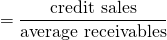Next, we have inventory turnover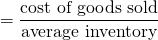Finally, we need the payables turnover ratio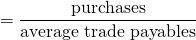Next, we divide 365 by the reciprocal of each of these variables. This gives us the average number of days of receivables, average number of days of inventory, and average number of days of payables, respectively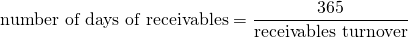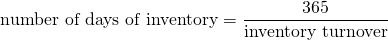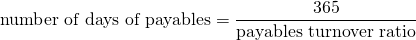Finally, now that we have the different components, the cash conversion ratio calculation equals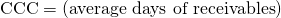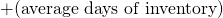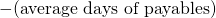## What is a good cash conversion cycle ratio?

This depends on the sector. Some sectors have very short CCCs, other companies have very long CCCs. Thus, it is not possible to compare the CCCs between companies in different sectors or industries. For example, the cash conversion cycle retail industry average will be higher than that of a company that sells consumer durables.

Is it possible to have a negative cash conversion cycle? Yes, this means that the number of days of payables is very high. Therefore, a negative CCC means that the number of days of payables exceeds the sum of the number of days of inventory and the number of days of receivables. A negative CCC indicates that the a company relies heavily on suppliers to finance its working capital or uses very little working capital to finance the first two components.

How to improve the cash conversion cycle? Clearly, we should lower the first two components, i.e. the number of days of receivables and the number of days of inventory, and increase the number of days of payables.

## Cash Conversion Cycle Example

The following figure provides a numerical example that illustrates the different steps needed to calculate the CCC. We first calculate the three necessary ratios separately and then combine them to come up with the CCC.